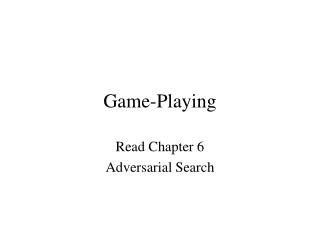DownloadDownload PresentationGame-Playing

# Game-Playing

Download Presentation## Game-Playing

- - - - - - - - - - - - - - - - - - - - - - - - - - - E N D - - - - - - - - - - - - - - - - - - - - - - - - - - -
##### Presentation Transcript

2. State-Space Model Modified • States the same • Operators depend on whose turn • Goal: As before: win or win amount • Search: somewhat different

3. Game Types • Two-person games vs multi-person • chess vs monopoly vs poker • Perfect Information vs Imperfect • checkers vs card games • Deterministic vs Non-deterministic • go vs backgammon

4. Simplest First:Two-Person Games: Perfect Information • BF = branching factor (average) • Chess: BF ~36 • expert level • Checkers: BF ~ 8, world champion • Othello: BF ~10, better world champion • Go: BF ~200 • \$2 million prize

5. MiniMax Algorithm (perfect information, 2 person game) • Assume: evaluation of terminal position • Win = +1, Loss = -1, Draw = 0. • Descendants of max node is min node, etc. • Algorithm: recursive • Value Max Node = max(descendants of node) • Value Min Node = min(descendants of node) • Value of terminal node: by evaluation function • Applies to any tree with values assigned to leaves. • NOTE: If tree to end of game, guaranteed best move, else no one knows.

6. MiniMax Example

7. Optimal Play • Make move that yields highest minimax score. • Computation: search: depth-first • Time = b^d • Memory= b*d

8. Applied to Chess • Average game is 40+ moves • Tree to large to reach terminal positions • Static board evaluation of worthiness • Uses Partial Tree • MiniMax yields optimal value for restricted tree, with values assigned by evaluation. • No theorems connecting valuation on partial tree to estimates for complete tree.

9. Alpha-Beta Algorithm • Yields exactly same value as minimax • Knuth analyzed: time or nodes = O(b^d/2) • Doubles depth of search with same time. • Constant depends on ordering of nodes • Iterative deepening alpha/beta achieves better ordering. (reorder after depth)

10. Alpha-beta Algorithm • Each node is assigned a range of values: [alpha,beta]. The real value will lie between. • The root is assigned [-inf,+inf]. • For any max node N with values [A,B] • if a son has value >=C, then N has new range [C,B]. • If interval is empty, all nodes below cut. • For any min node N with values [A,B] • if son has value <=D, then N updated to [A,D]. • Formal code in text. • http://www.cs.mcgill.ca/~cs251/OldCourses/1997/topic11/ • http://www.ocf.berkeley.edu/~yosenl/extras/alphabeta/alphabeta.html Applet illustration

11. Alpha-Beta Example

12. Alpha-Beta Example

13. (1,2,2) Nim

14. Multi-player Games • Extension of minimax • assign a vector of values to each position • vector has value relative to each player • Each player maximizes choice • Equals minimax for 2 person game • No variations like alpha-beta

15. Games with Uncertainty • Card games like hearts or bridge • Backgammon (roles of dice) • Expectimax • Does it work? • Theoretically nice, but where’s the meat – for what games was it successful?

16. Certainty from Uncertainty • Simulation • Replace unknown world by specific world • simulate (or use alpha-beta) • Each simulation yields a play • Vote • Works for hearts and bridge play • bridge high level card play can’t make information gathering plans

17. What about War • Games are games – restricted uncertainty • What are the operators in war? • unknown effects • unknown number • What is the state? • unknown